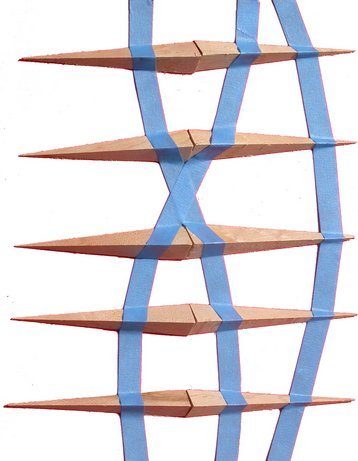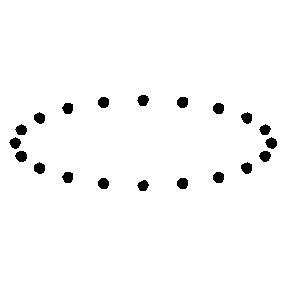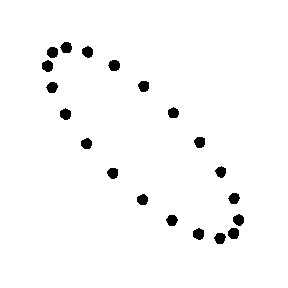[Contents]

Pre-Introduction to Gravitational Waves
John Denker

## 1  Overview

This is not meant to be a comprehensive tutorial. Instead the focus is on ideas that are (a) very basic and fundamental, and/or (b) not necessarily well explained elsewhere.

Most people can figure out that gravitational waves have something to do with gravitation and something to do with waves. They may have heard something about ripples in fabric of spacetime. However, if you want to understand gravitational waves in more detail than that, it requires a fairly deep understanding of waves, spacetime, and gravitation.

This is a fairly hot topic right now, partly because gravitational waves were recently observed for the first time, and because some of the leaders of the project were awarded the 2017 Nobel Prize in physics (reference 1). They had been working on it for 50 years. About a billion dollars was invested in the effort.

This is a very difficult topic! Einstein himself was confused by it. He was not sure whether gravitational waves had any real, observable effects. He changed his mind several times over the years. Later, Feynman came up with the Gedankenexperiment shown in figure 1. The beads are free to move in response to the gravitational wave, while the stick defines what we mean by distance, in a way that is mostly immune to the wave. For the next level of detail on this, see reference 2.Figure 1: Gravitational Wave Detector: Beads Sliding on a Stick

## 2  Electromagnetic Analogy

Arguing by analogy is always risky. Let’s start by discuss a few ways in which gravitational waves (G waves) are analogous to electromagnetic waves (EM waves). Later we will discuss ways in which the analogy breaks down.

1. The near field is a mess. Things are simpler in the far field. This is true for both G waves and EM waves. From now on, we will discuss the far field only, unless otherwise stated.
2. The ordinate of the wavefunction is a field. The field is transverse. That is, the effect of a field on a stationary test particle is perpendicular to the direction in which the wave is traveling. This is true for both G waves and EM waves.

This stands in contrast to sound waves in air or water, where the ordinate of the wavefunction is longitudinal (not transverse).

3. In the far field, for a wave spreading out from a compact source, the wavefronts are spherical, and the field strength is proportional to 1/r. This is true for both G waves and EM waves.
4. The previous item should make it obvious that you cannot understand EM waves starting from Coulomb’s law. The r-dependence is all wrong. Also the t-dependence is also wrong, although that is less obvious. Furthermore, the polarization (i.e. the direction of the associated force) is all wrong. The same issues affect EM waves and G waves alike. You cannot understand G waves starting from Newton’s law of universal gravitation.

Sure, you can wiggle the source and create wiggles in the Coulomb field, and similarly in the Newtonian gravitational field, but all that is confined to the near field, and tells you essentially nothing about what’s going on in the far field. Let’s be clear: Your intuition about wiggles in the Newtonian gravitational field is 100% wrong in region where properly-behaved waves exist, i.e. in the far field.

5. The energy per unit volume and the power per unit area are proportional field strength squared. This is true for EM waves and G waves alike.

In particular, for the aformentioned spherical waves, both energy per unit volume and power per unit area are proportional to 1/r2. This scaling law is exceedingly unsurprising, since the area of the sphere grows in proportion to r2, and we expect energy to be conserved.

6. The Maxwell equations contain Coulomb’s law as a special case, but they also contain other terms, and it is the other terms that explain EM waves. By the same token, Einstein’s field equations contain Newtonian gravity as a special case, valid in the near-field zero-frequency limit, but they also contain other terms, and it is these other terms that explain G waves.

## 3  Spacetime

Any serious discussion of gravitation in general (and G waves in particular) is based on general relativity. There is no hope of understanding general relativity unless you have a firm grasp of special relativity. Special relativity is the geometry and trigonometry of spacetime – nothing more and nothing less.

As you have presumably heard, time is the fourth dimension. That is a quantitative, formal, scientific idea. It should be taken very seriously. The timelike dimension is not exactly the same as the spacelike dimensions, but it is more similar than it is different. This is a tremendous advantage, because it means that most of your intuition about geometry and trigonometry can be carried over from Euclidean space to Minkowski spacetime, with only minor modifications here and there.

For details on this, see reference 3.

## 4  Gravity

The word gravity (or equivalently gravitation) has two different meanings. This is a mess, because typical textbooks do not explain the distinction. They switch back and forth without warning.

• One concept of gravity is the local gravitational acceleration g, relative to some chosen reference frame. We call this the framative gravity. One example is the ordinary gravity experienced by terrestrial pedestrians. This is completely dependent on the choice of reference frame; the acceleration experienced by astronauts aboard the space station is either approximately 88% of the ordinary terrestrial gravity (if you use a terrestrial reference frame) – or it is thousands of times smaller than that (if you use a frame comoving with the space station).

The laws of physics correctly describe what happens, no matter what reference frame (if any) you choose.

• The other concept of gravity is completely independent of the choice of reference frame. It depends on the distribution of mass, in accordance with Newton’s law of universal gravitation. We call this the massogenic gravity. It explains the difference in g between Spain (on one side of the earth) and New Zealand (on the other side). You can change g in one place or the other by choosing a reference frame, but you cannot change the difference.

To say the same thing another way, this place-to-place variation in g gives rise to what we call the tidal stress.

The next level of detail on these concepts, see reference 4.

This is crucial to understanding G waves, since they are not waves in the g field, but rather waves in the tidal stress.

## 5  Spacetime Curvature

Framative gravity has got nothing to do with spacetime curvature. A uniform g field corresponds to zero curvature. You can find lots of articles and diagrams that purport to explain g directly in terms of curvature, but they are all hogwash.

Figure 2 correctly shows how spacetime curvature can give rise to tidal stress. For an explanation, see reference 2.Figure 2: Orbits due to Curvature due to Darts

## 6  Polarization

To explain what we mean by polarization, we show diagrams of what happens to an array of test particles acted upon by a plane wave. (The wave is propagating in the direction perpendicular to the plane of the diagram.)

The given polarization states are of course not the only possibilities. Any linear combination of polarization states is also a polarization state. Also any phase-shifted state. Also any linear combination of phase-shifted states.

Two linearly-independent polarizations of a G wave are shown in figure 3 and figure 4. These two states differ by a 45 degree rotation, which is different from what you see in EM waves.Figure 3: G Wave Plus Polarization Figure 4: G Wave Cross Polarization

Two linearly-independent polarizations for EM waves are shown in figure 5 and figure 6. The two states differ by a 90 degree rotation.Figure 5: EM Wave X Polarization Figure 6: EM Wave Y Polarization

## 7  Wave Mechanics

G waves propagate at the speed of light, denoted c.

G waves play by the same basic rules as any other waves. For example, for a monochromatic wave, the frequency times the wavelength is the wave speed (c). High frequencies correspond to short wavelengths.

The events that the LIGO detector has been seeing produce frequencies in the range from 30 Hz up to 1 kHz or thereabouts. That corresponds to ITU frequency bands 2 and 3, i.e. SLF and ULF. That’s 100 times lower than AM radio frequencies, but about 10 times higher than the ELF frequencies that the US Navy uses for sending radio signals to submarines.

A 1 kHz frequency corresponds to a wavelength of 300 kilometers. So you can understand why the LIGO arms are 4 km long. To a first approximation, the longer the arm the better it is able to pick up the signal. However, anything much longer than 4 km would be impractical. Given that arm length, you can understand why the arm cavity has a finesse on the order of 200. To a first approximation, the more round trips the light makes, the better it is able to pick up the signal. That finesse requires astonishingly good mirrors.

On the other hand, further improvements in the mirrors wouldn’t help, especially at the high end of the frequency range, because it would mean that the instrument was still responding to the previous cycle while a new cycle was starting.

## 8  Quantum Mechanics

The universe in which we live is quantum mechanical. The laws of quantum mechanics always apply. Quantum mechanics will tell you if-and-when the various classical approximations are valid, but even then, the QM description is always valid and always more fundamental. By the way, the converse does not hold: Classical mechanics knows almost nothing about the limits to its own validity that are imposed by quantum mechanics.

The quanta of a G wave are called gravitons. The corresponding quanta of an EM wave are called photons.

Provided you have a monochromatic wave at frequency ω and provided you measure the energy thereof, you will find that the energy is quantized in units of ℏω. This applies to G waves and EM waves and everything else. Quantum mechanics allows no wiggle room on this.

Beware that there are two very important provisos at the start of the previous paragraph. If you measure an EM wave with a photon counter, you will observe an integer number of photons ... but if you measure it with a voltmeter, the voltage will not be quantized. The voltage will not be restricted to integer multiples of anything. See reference 5. Einstein got this wrong. He assumed that the EM field was always quantized, but it most certainly is not. You may choose to measure it with a photon counter, but that’s a choice, not a law of nature. A great many textbooks get this wrong, at the introductory level and several levels beyond that.

LIGO is not a graviton counter. It measures field strength directly, so it is more akin to a voltmeter than to a photon counter. This is actually quite important, because the number of quanta scales like the square of the field strength; this applies to photon numbers and graviton numbers alike. When the field strength is small, the number of quanta is small squared, which is not helpful. A graviton-counting instrument would have horrifically bad sensitivity.

The graviton is a spin-2 particle. This stands in contrast to the photon, which is a spin-1 particle. You can begin to understand what this means as follows: Imagine waves of water sloshing in a circular tub, as discussed in reference 6. A |2px⟩ wavefunction means the water is sloshing back and forth in the x-direction, like a see-saw. This corresponds to figure 5.

If you rotate your head 90 degrees, a |2px⟩ wavefunction turns into a |2py⟩ wavefunction. If you start again with the original |2px⟩ wavefunction and turn your head 180 degrees, you get a −|2px⟩ wavefunction, including a minus sign. If you start again and turn your head 360 degrees, you get back the |2px⟩ wavefunction completely unchanged. In other words, the wavefunction undergoes one full cycle of phase change as you go around the circumference of the tub. The |2p+⟩ wavefunction, which is a linear combination of |2px⟩ and |2py⟩ (with a phase shift) has 1 unit of angular momentum. This is how EM waves behave.

In contrast, the wave in figure 3 corresponds to a |3dx2y2⟩ wavefunction. If you turn your head 90 degrees you get the same wavefunction with a minus sign, and if you turn your head 180 degrees you get back the original wave function completely unchanged. In other words, the wavefunction undergoes two full cycles of phase change as you go around the circumference of the tub. A linear combination of plus-polarization and cross-polarization (with a phase shift) has 2 units of angular momentum. This is how G waves behave.

This discussion of quanta is super-theoretical, because nobody is going to build a graviton counter anytime soon.

## 9  Some Terminology

1. The name LIGO is an acronym: Laser Interferometer Gravitational-Wave Observatory. I’m not sure what happened to the “W”.
2. The name of the Virgo Collaboration is not an acronym, and it is commonly written in non-all-caps form, as I just did.

It is named after the Virgo cluster of galaxies, of which the local group is a member. The cluster is in turn named for the constellation Virgo, since the center of the cluster is in that part of our sky.

The name is significant, since the current generation of instruments was designed to detect the coalescence of a compact binary star system anywhere within the Virgo cluster. You have to look at a volume of that magnitude if you want to see coalescence events at a reasonable rate.

## 10  References

1.
“The Nobel Prize in Physics 2017”
https://www.nobelprize.org/nobel_prizes/physics/laureates/2017/
https://www.ligo.caltech.edu/page/press-release-2017-nobel-prize
2.
John Denker,
“Tabletop Geodesics, General Relativity, and Embedding Diagrams”
www.av8n.com/physics/geodesics.htm
3.
John Denker,
“Welcome to Spacetime”
www.av8n.com/physics/spacetime-welcome.htm
4.
John Denker,
“Weight, Gravitational Force, Gravity, g, Latitude, et cetera”
www.av8n.com/physics/weight.htm
5.
John Denker,
“Coherent States”
www.av8n.com/physics/coherent-states.htm
6.
John Denker,
“Models and Pictures of Atomic Wavefunctions”
www.av8n.com/physics/wavefunctions.htm
[Contents]